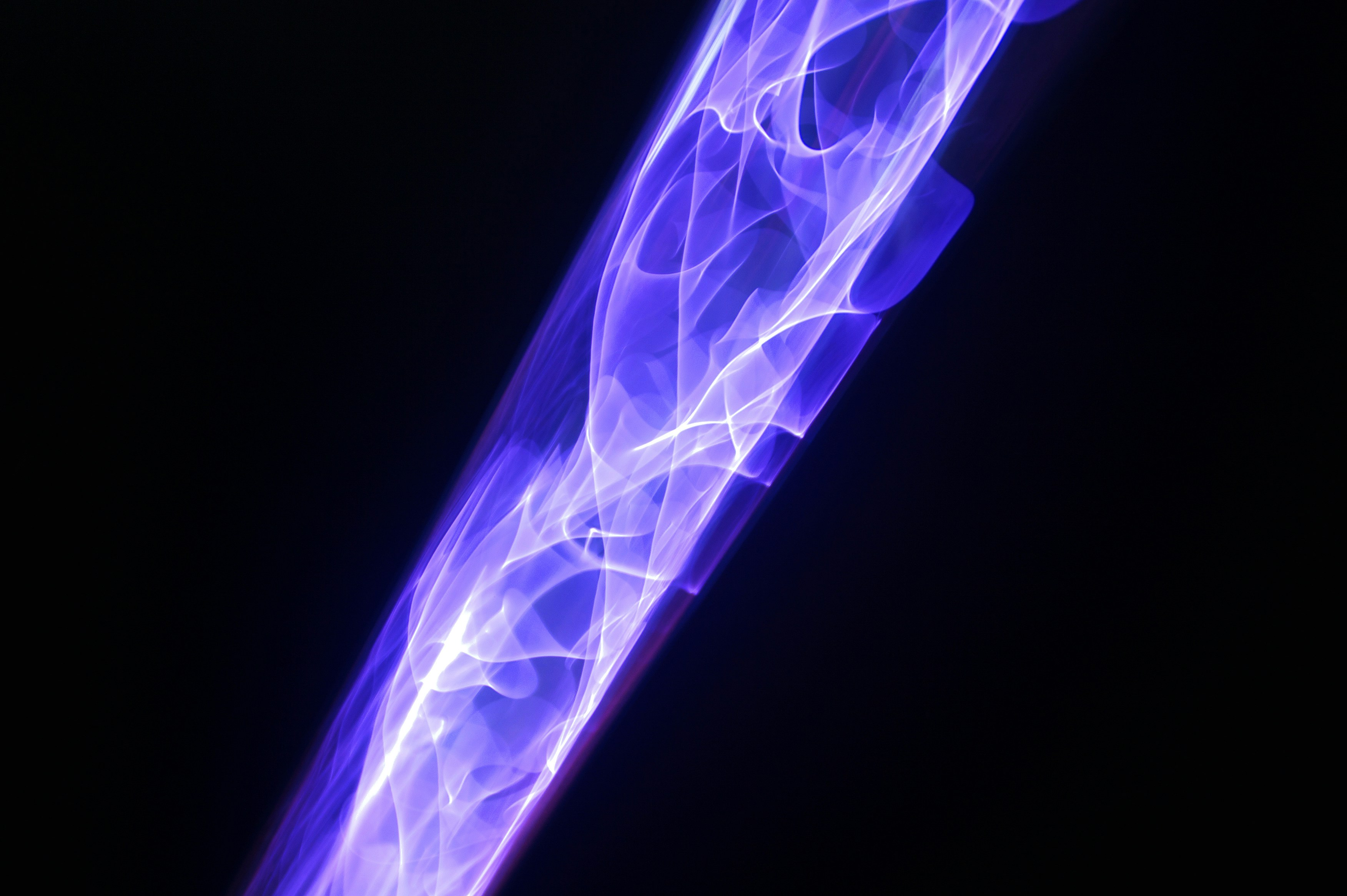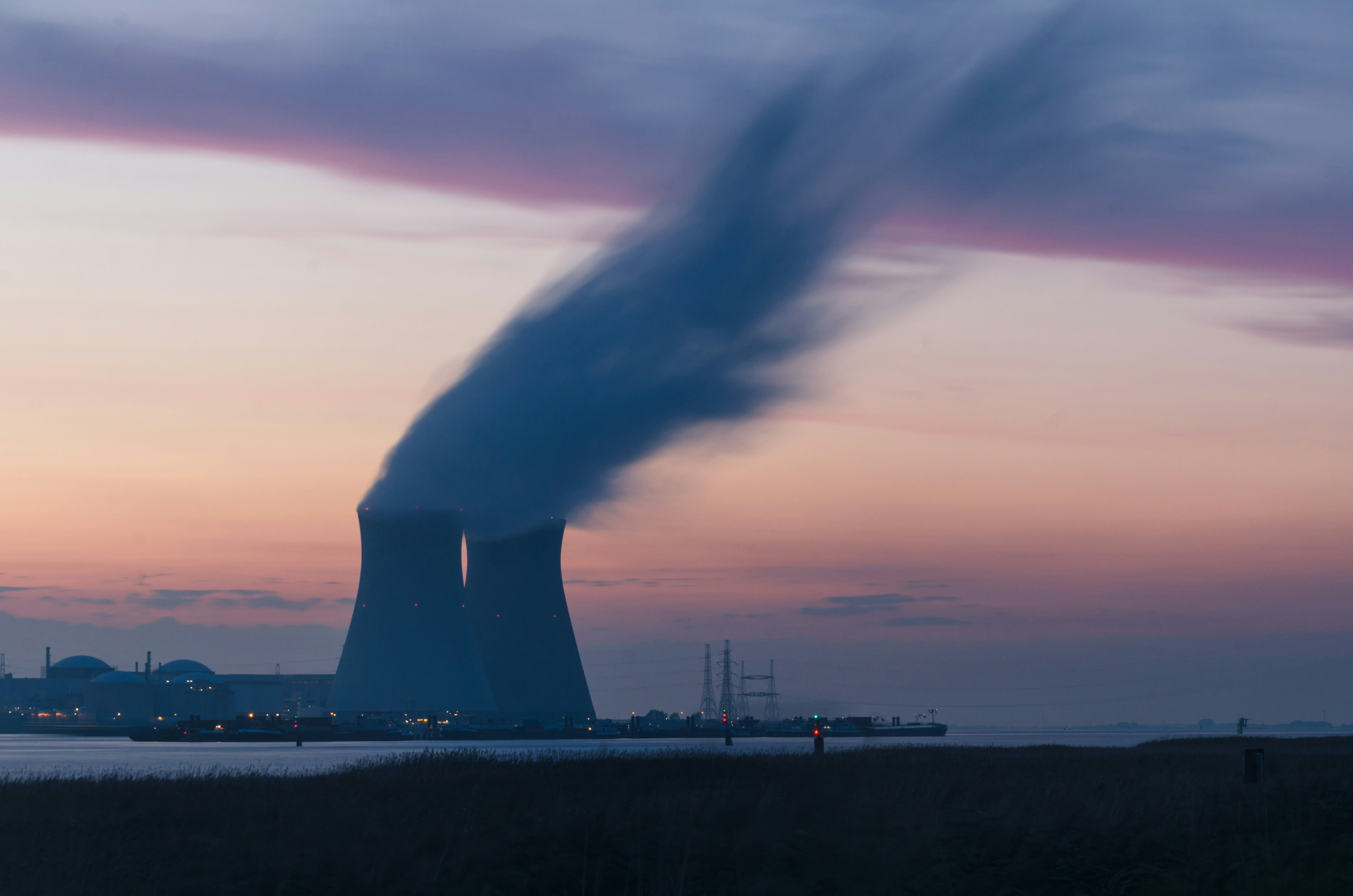# Richard Vasques

Assistant Professor of Nuclear Engineering

# ResearchMathematical and computational modeling of particle transport in systems where the Beer-Lambert law fails to hold and nonexponential attenuation takes place. Applications in atmospheric clouds, reactor physics, medical imaging, and computer graphics.Accelerating the convergence of the solution of transport problems with highly forward-peaked scattering using a modified Fokker-Planck equation.Novel mathematical model and computer simulation environment to realistically predict the behavior of recurrent tumors undergoing combined-hyperthermia-radiotherapy (CHR) treatment.Modeling of particle transport taking place in random media, using approaches such as the Levermore-Pomraning equations and the Atomic Mix Model. Applications include astrophysics, nuclear medicine, reactor physics, and atmospheric sciences.Studying the influence of stochastic fluctuations upon the results of the neutron point kinetics equations and finding an approach to obtain a nonstiff solution for the stochastic neutron point kinetics equations.The goal of this project - undertaken in cooperation by RWTH Aachen University, Forschungszentrum Jülich, and SIEMENS AG - was to study the feasibility of a compact neutron imaging system for radioactive waste inspection.This project focused on the implementation and improvement of numerical solvers for the transport equation using Laplace transforms. Approaches included the LTSn and LTAn methods.

Share